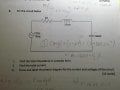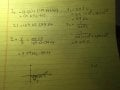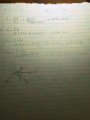# AC circuits phasor diagram

#### Yami

Joined Jan 18, 2016
262
Hi I'm having some confusion drawing the phasor diagrams for the current and voltage. Did some research on the net and the text book. Still I'm confused. Can't one draw the V and I with the phase angle difference. Does it have to be a certain way for series circuit and another way for parallel circuits? and my teacher was not very clear about this when I asked him. He mentioned something about the impedance diagram which added to the confusion even more :S.
I've included the question and the way I worked it out.
Ignore the work done on the question paper.#### Yami

Joined Jan 18, 2016
262
And here is the parallel circuit question with the sin wave drawn. Am I right on how I changed to the time domain?. and is it right with the phase angle difference?

#### DGElder

Joined Apr 3, 2016
351
Hi I'm having some confusion drawing the phasor diagrams for the current and voltage. Did some research on the net and the text book. Still I'm confused. Can't one draw the V and I with the phase angle difference. Does it have to be a certain way for series circuit and another way for parallel circuits? and my teacher was not very clear about this when I asked him. He mentioned something about the impedance diagram which added to the confusion even more :S.
I've included the question and the way I worked it out.
Ignore the work done on the question paper.

View attachment 114156 View attachment 114157
Your Zt is correct. Though, I am not sure exactly what they mean by "complex form".
I interpreted that to mean complex cartesian form as opposed to polar form. Generally, it is easier to add impedances in cartesian form when the lumped elements are complex impedances of differing phase angles and not just imaginary and real values -which is not the case here. In any case your final answer is correct.

The current you have calculated and the phase diagram is correct for the source (though you need to label it with the current magnitude). So just continue this for each of the other elements - voltage = I*Z. You have already drawn the current phasor you just need to show that and the labeled voltage phasor for each element. Do you know how to multiply phasors: multiply magnitudes and add the phase angles? So the angle between the current and voltage vectors for the passive elements is going to be either zero or +/- 90 degrees.

Last edited:
•Yami

#### DGElder

Joined Apr 3, 2016
351
And here is the parallel circuit question with the sin wave drawn. Am I right on how I changed to the time domain?. and is it right with the phase angle difference?

Yes, your approach is correct, your sketch is correct and your numbers are at least close - I didn't check the arithmetic. But label your units! Also displaying your answer with 5 significant digits implies a high level of accuracy and resolution that does not exists in the problem nor in real world power circuit problems for that matter. The known impedances in this problem are only given with 2 significant digits - at best.

Last edited:
•Yami

#### shteii01

Joined Feb 19, 2010
4,644
Complex form tells me that they want it in a+bj form. Your answer is not in this form, I expect they will take points off for that.

#### Yami

Joined Jan 18, 2016
262
Thanks so much for your help. Good point about the complex form - its just easily convertible from the calculator. So should I label the current magnitude in the positive Y axis? and on question 2 I showed is my approach on drawing the sin wave ok? Change it to time domain and then sketching the curve. I did another similar question too. I've attached it.
Also there was another question which had RLC all in parallel. The individual reactance and resistance were given, but for the inductor it was labeled that 1 amp was in that branch. The source voltage was given. So I know the value of the voltage and I could use the ohms law to find the indcutive reactance- my question is that would you have to use the angles when finding it?or could I use the magitude of the values given.
And also with power factor how do you know whether its leading or lagging?

#### DGElder

Joined Apr 3, 2016
351
So should I label the current magnitude in the positive Y axis? and on question 2 I showed is my approach on drawing the sin wave ok? Change it to time domain and then sketching the curve.
See my EDITS:

So should I label the current magnitude in the positive Y axis?

No, the vertical axis does not represent current; it is the imaginary axis of the complex plane upon which both current and voltage phasors are drawn. Actually you did label the current phasor symbolically which is fine since you indicate the magnitude elsewhere. Note of caution, be sure it is clear whether you are drawing with rms or peak values for your phasors. In power analysis rms or effective values are used but otherwise peak values are usually used. The time domain representation of a phasor is the projection of the anticlockwise rotating vector onto the x (real) axis (which won't work with an rms phasor magnitude). Appropriate magnitude representation depends on what you are doing.

While your phasor sketch is OK, it is customary to use the current as the reference phasor when you are diagramming the phase relationship of elements that are in series - since they all share the same current. So it would be better to show the current at 0 deg phase and show all the voltages with respect to that. In a parallel circuit you customarily use the voltage as the reference phasor since all the branches share the same voltage.

on question 2 I showed is my approach on drawing the sin wave ok? Change it to time domain and then sketching the curve

Your time domain sketch correctly shows the relationship between current and voltage. However, technically, the time domain representation of a phasor is the real portion of the exponential function |R|*e^j(wt+theta) - where |R| is the magnitude of the voltage or current phasor R. Using Eulers identity to transform that to a sinusoidal function and taking only the real part, the time domain representation would be |R|Cos(wt + theta). So, for example, here, v = 140 cos(wt +2.3deg).

So is your answer wrong? I would say no (your instructor may disagree), since the original problem does not explicitly peg the voltage source signal to a specific point in time, i.e. the voltage at t=0 is ambiguous - though it might be implied by the context of the problem inside your study of phasors. Sticking to the cosine function or a 90 deg shift in phase with a sin function is safer.

Last edited:
•Yami

#### DGElder

Joined Apr 3, 2016
351
Thanks so much for your help. Good point about the complex form - its just easily convertible from the calculator. So should I label the current magnitude in the positive Y axis? and on question 2 I showed is my approach on drawing the sin wave ok? Change it to time domain and then sketching the curve. I did another similar question too. I've attached it.
Also there was another question which had RLC all in parallel. The individual reactance and resistance were given, but for the inductor it was labeled that 1 amp was in that branch. The source voltage was given. So I know the value of the voltage and I could use the ohms law to find the indcutive reactance- my question is that would you have to use the angles when finding it?or could I use the magitude of the values given.
And also with power factor how do you know whether its leading or lagging?

I did another similar question too. I've attached it.

You have the wrong sign for the complex power angle. P = (Veff) (I*eff) = Veff * Ieff * e^j(Vtheta - Itheta). And again you show too many significant digits in your answer and don't label the units. Also, I imagine your instructor expects you to clearly differentiate in some way between phasors and scalar values. You are just using capital letters for both. You might indicate phasors with a ^ on top or something like that to distinguish it from a scalar quantity such as the magnitude of the phasor.

would you have to use the angles when finding it?or could I use the magitude of the values given

To find what? Show the problem.

And also with power factor how do you know whether its leading or lagging?

See my post below. [edit 10/28]

Last edited:

#### Yami

Joined Jan 18, 2016
262
Thanks again for the help. I have redone the the phasor diagram for Q1, included all the voltages. The question now is what if the source voltage has got an angle because so far the question had the voltage at 0 degrees.
The reason how I drew the way before was, in my head it was asking for the source voltage and total current phase difference.

I've attched the question about the parallel AC circuit and showed how I calulated the inductive reactance of the inductor. My question was since the angle of the current was not given would I have to use 0 degree or not use any angle at all?

Regarding the power factor :- Tell me if I got this correctly
eg : (some arbirtary numbers from the top my head)

1) V<0°
I < 53.13°
power factor = cos(θV-θI) = cos(-53.13) =====>since its a negative angle its lagging? and if it were a postive angle its leading?

2) V<53.13°
I<0°
power factor = cos(53.13)===== leading because angle is positive?

#### DGElder

Joined Apr 3, 2016
351
Regarding the power factor :- Tell me if I got this correctly
eg : (some arbirtary numbers from the top my head)

1) V<0°
I < 53.13°
power factor = cos(θV-θI) = cos(-53.13) =====>since its a negative angle its lagging? and if it were a postive angle its leading?

2) V<53.13°
I<0°
power factor = cos(53.13)===== leading because angle is positive?

Sorry, that is not correct. My fault, I mislead you.

If the voltage leads the current then the complex power angle is positive, but the power factor is said to be lagging.

You can think of it this way:

Real power leads the apparent power

For a lagging power factor:
Current lags the voltage
Real power lags the apparent power.

So for example:
V /_ 0 deg.
I /_ 53 deg.

Last edited:
•Yami

#### Yami

Joined Jan 18, 2016
262
Ahh I see now. Going back to the question with series and parallel components how would I draw the phasor diagram when it has got series and parallel components. Is my approach right? Im referring to #6 post up

#### DGElder

Joined Apr 3, 2016
351
Ahh I see now. Going back to the question with series and parallel components how would I draw the phasor diagram when it has got series and parallel components. Is my approach right? Im referring to #6 post up
If you are drawing phasors to establish instantaneous V and I's, then you must tie the phase to the time domain and therefore the phases are not arbitrary. Otherwise...

Phasors show the relative phase relationship between Vs and Is in the frequency domain in a steady state circuit. This relationship can be established using any of the phasors as the reference, /_0, or none. As long as the relative magnitudes of the currents to each other and the relative magnitude of the voltages to each other and the phase relationships are preserved.

There is no wrong reference, but there are better references - it is a matter of judgement and exactly what you are trying to analyze or communicate and choosing the simplist or clearest diagram to that end. Generally, if there is no clear preferred reference phasor I would make the source voltage the reference.

Now if the problem statement, as in post #6, establishes a particular phase - in this case V/_0 for the source, then you should stick to that phase. So, here, use the voltage of your source as your reference phasor. Likewise if the problem statement expresses the signal in the time domain, for example E=Vcos(wt+theta) then strictly the phasor diagram should maintain that phase, theta, for E. But again, depending on what you are trying to do, it may be easier to set that phase or a current phase at 0 degrees as long as you make clear you have broken the phase relationship with the time domain signal. It depends on context and intent.

That's my opinion. You might ask your instructor if he has a more explicit protocol since he is grading your papers. His/Her priority might be on ease of grading papers - lol.

P.S. your V phasor should be 120V not 100V.

Last edited: